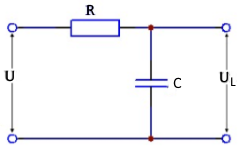# R/C for given charging voltage

Calculate R/C for the charging voltage at a given time"

## R/C to calculate a charging voltage

On this page you can calculate the values of a capacitor or resistor that are required to achieve a certain charging voltage on a capacitor at a given point in time.

Use the radio button to select whether the resistance or the capacitor should be calculated and enter the values. Then click on the 'Calculate' button.

Calculate R/C to charging voltage

 What should be calculated? Resistor R Capacitor C Input Capacitor F mF µF nF pF Resistor mΩ Ω kΩ MΩ Voltage U mV V kV Voltage UL mV V kV Loading time nSec µSec mSec Sec Decimal places 0 1 2 3 4 6 8 10 Resultat Rasistor Capacitor
 $$\displaystyle R$$ Resistor $$\displaystyle C$$ Capacitor $$\displaystyle τ$$ Time constant $$\displaystyle t$$ Loading time $$\displaystyle U$$ Source voltage $$\displaystyle U_C$$ Capacitor loading voltage## Formulas vor calculation of R and C

$$\displaystyle C = \frac{t·(-1)}{R ·ln\left(1-\frac{U_C}{U}\right)}$$

$$\displaystyle R= \frac{t·(-1)}{C ·ln\left(1-\frac{U_C}{U}\right)}$$